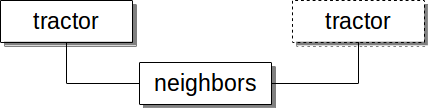# 4.1. Example Queries¶

## 4.1.2. Neighbors¶

Construction in Progress: The neighbors table is under construction but the principles shown here are still useful enough to publish.

Some databases may have a precalculated neighbors table. Here, the neighbors table includes all neighboring objects within 30 arcsec. To use it, we will need to to perform two JOINS. The neighbors table does not provide attributes such as flux, but it does have a key on objid. Also, since we are narrowing the Ra, Dec range to within a brick, there’s no need to indicate the

Todo

finish previous sentence

SELECT  t1.flux_g / t1.flux_r -  t2.flux_g / t2.flux_r as rng --Difference between source and neighbor's g-r magnitude,
n.dist_arcsec,                                        --Distance of neighbor
t1.brickid,  t1.objid as source_objid,                --Source unique identifier (BRICK,OBJID)
t1.flux_g/ t1.flux_r as source_g_r,                   --Source g-r magnitude
t1.shapeexp_r as source_shapeexp_r,                   --Source Half-light radius of exponential model (>0) arcsec
t1.shapeexp_e1 as source_shapeexp_e1,                 --Source Ellipticity component 1
t1.shapeexp_e2 as source_shapeexp_e2,                 --Source Ellipticity component 2
t2.brickidk, t2.objid as neigh_objid,                 --Neighbor unique identifier (BRICK, OBJID)
t2.flux_g/ t2.flux_r as neigh_g_r,                    --Neighbor g-r magnitude
t2.shapeexp_r as neigh_shapeexp_r,                    --Neighbor Half-light radius of exponential model (>0) arcsec
t2.shapeexp_e1 as neigh_shapeexp_e1,                  --Neighbor Ellipticity component 1
t2.shapeexp_e2 as neigh_shapeexp_e1                   --Neighbor Ellipticity component 2
FROM  tractor t1
INNER JOIN ls_dr3.neighbors n
ON t1.objid = n.obj_id
INNER JOIN  ls_dr3.tractor t2
ON t2.objid = n.neighbor_id
WHERE (t1.ra BETWEEN 153.7561 and 153.8882) and (t1.dec BETWEEN SYMMETRIC  0.6250 and 0.6792)
and (t2.ra BETWEEN 153.7561 and 153.8882) and (t2.dec BETWEEN SYMMETRIC  0.6250 and 0.6792)
and t1.flux_r > 0                                      --To calc magnitude we exclude 0s to avoid divide by 0
and t2.flux_r > 0
and t2.objid != t1.objid                               --No need to compare an object with itself
ORDER BY rng, n.dist_arcsec DESC
LIMIT 2;


Returns 2 rows in ~ 1.8 sec

We inner join tractor and neighbors and then we inner join neighbors to tractor aliased under a different name. Note how we alias the first tractor with t1 and the second tractor table with t2. You may use any alias you wish but keep them distinct.

For the Ra and Dec bounds we have to specify them twice, one for each reference to tractor. If we did not include the bounds for t2, the database would scan all of t2, taking a very long time. If you were using a viewer to find boundaries to search, this query might be how you search the range.

When using the BETWEEN operator, the first operand must be smaller than the second one, otherwise you will not get a match. To support ranges without regard to order add the SYMMETRIC keyword as shown above.Todo

image is missing

## 4.1.3. Cross Match¶

Cross matching is a resource-intensive operation, so expect it to run quite long. However, you can get really good performance if you have one table significantly small in number of rows.

Here we have the Dark Energy Survey Science Verification first Annual survey cross-matching against the GAIA DR1 survey within .001 degrees.

Note here we do not use the JOIN clause in SQL which is one reason it is a resource-intensive query. This is the time you want a Cartesian join due to the nature of cross-matching. The other thing to notice is the smaller table by number of rows is listed first in the q3c_join() clause (i.e. d.ra_j200 and d.dec_j2000).

SELECT count(*)
FROM des_sva1.gold_catalog d,
gaia_dr1.gaia_source g
WHERE q3c_join(d.ra_j2000, d.dec_j2000, g.ra, g.dec, .001);


Returns 1,307,402 rows in 179 sec.